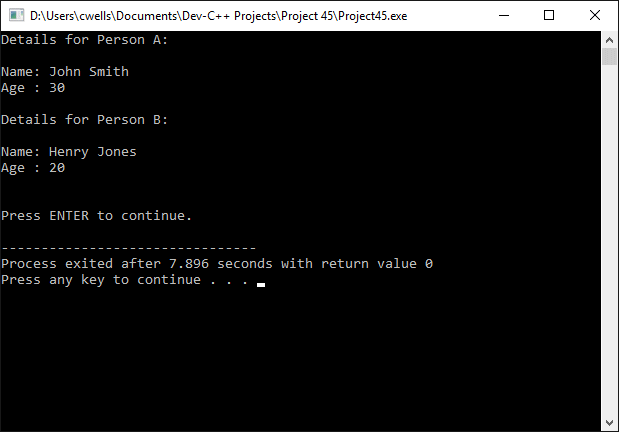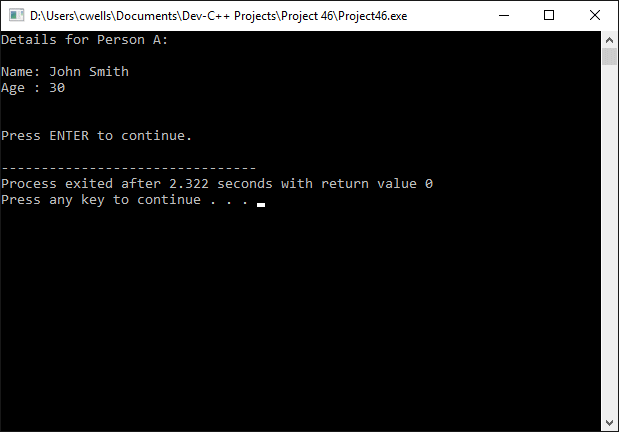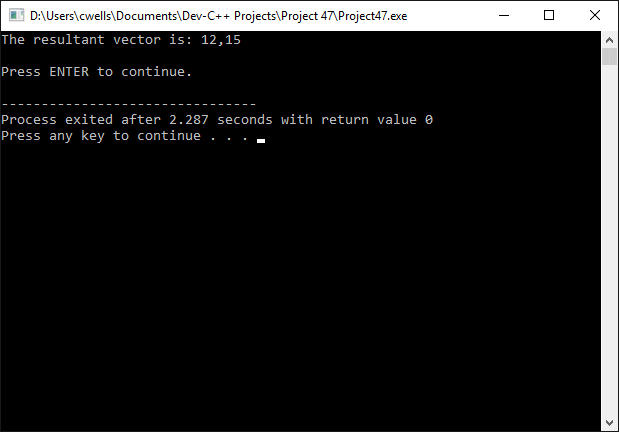# Introduction to Classes

Class objects in C++ are similar to structures, with some important differences. Like structures, they encapsulate a number of data items that can have different data types (including structure types or even other class types). Unlike structures, they also encapsulate functions (known as methods) that manipulate the class data members. The details of the data structures used and the implementation of the methods defined are hidden inside the object. All the programmer needs to know is what tasks the object can perform, and the parameters required by the methods provided. Essentially, a class is a template from which an object is created, and specifies the properties and methods that will be common to all objects that are created from it. An object, conversely, is an instance of a class.

Two (or more) different classes may have the same name, provide the same methods, and carry out the same tasks, even though they implement their functionality in different ways. The occurrence of two or more classes that have the same name and functionality but a different implementation is called polymorphism.

## Declaring a class

Classes are declared using the class keyword, as shown in the example below:

class person
{
public:
string name;
int age;
};

In this simple example, name and age are member variables of the class person. The public keyword is used to declare these member variables as being publicly accessible. Although the default access is private, we do not currently have any methods in place to return or modify the values they hold. The following program demonstrates how the person class might be used:

// Example Program 1

#include <string.h>
#include <iostream>
using namespace std;

class person
{
public:
string name;
int age;
};

string input_str;

int main()
{
person person_a, person_b;
person_a.name = "John Smith";
person_b.name = "Henry Jones";
person_a.age = 30;
person_b.age = 20;
cout << "Details for Person A:\n\n";
cout << "Name: " << person_a.name << "\n";
cout << "Age : " << person_a. age << "\n\n";
cout << "Details for Person B:\n\n";
cout << "Name: " << person_b.name << "\n";
cout << "Age : " << person_b. age << "\n\n";

cout << "\nPress ENTER to continue.";
getline( cin, input_str );
return 0;
}The output from example program 1

Normally, the member variables within a class are private (unless declared otherwise), and can only be accessed using the methods provided for this purpose by the class. The methods used to return or modify member variables are sometimes referred to as accessor methods. The other methods commonly found within a class are constructors and destructors.

## Constructors

A constructor function is called automatically when a class object is created, and its purpose is to initialise some or all of the class member variables. The person class that we saw previously can be modified to include a constructor function as follows:

class person
{
public:
string name;
int age;
person (string str, int n) {name = str; age = n;}
};

Note that the constructor function must take the same name as the class itself, and cannot have a return type. Note also that a constructor cannot be called explicitly, and is only executed when a new object of its class is created. The following statement creates an instance of the person class and calls the constructor function with appropriate parameters to initialise its member variables:

person person_a ("John Smith", 30);

The constructor can also assign default values to member variables, as in the following example:

class person
{
public:
string name;
int age;
person () {name = ""; age = 0;}
};

Like other functions, a constructor can be overloaded. The constructor called when an object is created will be the one that matches the parameters provided within the object declaration. The following code fragment includes a class declaration that includes both of the constructors previously used, together with examples of their use:

class person
{
public:
string name;
int age;
person (string str, int n) {name = str; age = n;}
person () {name = ""; age = 0;}
};
.
.
.
person person_a ("John Smith", 30);
person person_b;

In this example, person_b is declared without parameters, and will be initialised with the constructor that has an empty parameter list. Note that parentheses are not required for the declaration of an object that uses a constructor without parameters.

Note that if you do not include a constructor in a class definition, a default constructor is used that takes no parameters. Also by default, each class will include an implicit copy constructor and copy assignment operator (used to copy the member variables from another object to the current object), and a default destructor if none is explicitly defined (we will not be discussing the copy constructor and copy assignment operator here).

## Destructors

A destructor function is called automatically when an object is destroyed, either because the scope within which the object was declared has ended or, if the object was created dynamically, it is released using the delete operator. Like the constructor function, the destructor function takes the same name as the class and does not have a return type. Unlike the constructor function, however, the destructor is prefixed with the tilde character (~). Here is the previous declaration of our person class, this time with the destructor function included:

class person
{
public:
string name;
int age;
person () {name = ""; age = 0;}
~person (){name = ""; age = 0;}
};

Notice that in the relatively trivial example given, the functionality of the destructor is actually identical to that of the destructor.

## Accessor functions

The type of access given to member variables and methods can be explicitly determined using the keywords public, protected and private. These access specifiers as they are called work as follows:

• private - class members are accessible only to other members of the same class or to their friends (the topic of friendship will be dealt with elsewhere).
• protected - class members are accessible to other members of the same class, to their friends, and to derived classes.
• public - members are accessible to any part of the program within the same scope as the class object.

Note that by default, member variables are private, and once initialised their value can only be returned or modified by the accessor methods defined for the class. The following version of our person class declaration includes accessor methods to return and modify the member variables:

class person
{
string name;
int age;
public:
person () {name = ""; age = 0;}
~person (){name = ""; age = 0;}
void set_name(string str) {name = str;}
void set_age(int n) {age = n;}
string get_name() {return = name;}
int get_age() {return = age;}
};

The following program illustrates the use of the accessor methods defined for the person class:

// Example Program 2

#include <string.h>
#include <iostream>
using namespace std;

class person
{
string name;
int age;
public:
person () {name = ""; age = 0;}
~person (){name = ""; age = 0;}
void set_name(string str) {name = str;}
void set_age(int n) {age = n;}
string get_name() {return name;}
int get_age() {return age;}
};

string input_str;

int main()
{
person person_a;
person_a.set_name("John Smith");
person_a.set_age(30);
cout << "Details for Person A:\n\n";
cout << "Name: " << person_a.get_name() << "\n";
cout << "Age : " << person_a.get_age() << "\n\n";

cout << "\nPress ENTER to continue.";
getline( cin, input_str );
return 0;
}The output from example program 2

You can overload standard operators within a class definition. To do this, you declare operator functions, which take as their name the operator keyword followed by the symbol for the operator that you want to overload. In the following short program, the addition operator (+) is over-ridden to enable it to add two two-dimensional vectors to produce a resultant vector:

// Example Program 3

#include <string.h>
#include <iostream>
using namespace std;

class vector
{
public:
int x, y;
vector () {};
vector (int a, int b) { x = a; y = b;}
vector operator+ (vector v);
};

vector vector::operator+(vector v)
{
vector w;
w.x = x + v.x;
w.y = y + v.y;
return (w);
}

string input_str;

int main ()
{
vector a (4, 5);
vector b (8, 10);
vector c;
c = a + b;
cout << "The resultant vector is: " << c.x << "," << c.y;
cout << "\n\nPress ENTER to continue.";
getline( cin, input_str );
return 0;
}The output from example program 3

Note a few things about the above example program. First of all, we are going to use a variable of the type vector in the overloaded operator function, itself a member of that class. For this reason, we must define the function externally to the class declaration. Note also that we have created two constructors, one of which requires initial values to be passed to it for the x and y vector coordinates, and one that has a blank parameter list.

Note also the syntax used in the first line of the overloaded operator function:

vector vector::operator+(vector v)

Here the scope resolution operator (::) is used to identify the operator+ function as being a member function of the class vector.

## The this keyword

The this keyword represents a pointer to the object in whose member function it appears. The following program demonstrates the (admittedly somewhat superfluous) use of the this keyword:

// Example Program 4

#include <string.h>
#include <iostream>
using namespace std;

class Cuboid {
public:
Cuboid(double l, double w, double h) // constructor
{
length = l;
width = w;
height = h;
}

double volume()
{
return length * width * height;
}

bool compare(Cuboid cuboid)
{
return this->volume() > cuboid.volume();
}

private:
double length;
double width;
double height;
};

string input_str;

int main(void)
{
double L1 = 2.4;
double W1 = 4.7;
double H1 = 7.1;
double L2 = 1.6;
double W2 = 5.8;
double H2 = 7.3;

cout << "\nCuboid1: L=" << L1 << ", W=" << W1 << ", H=" << H1;
cout << "\nCuboid2: L=" << L2 << ", W=" << W2 << ", H=" << H2;

Cuboid Cuboid01(L1, W1, H1);
Cuboid Cuboid02(L2, W2, H2);

if(Cuboid01.compare(Cuboid02))
{
cout << "\n\nVolume of Cuboid2 < volume of Cuboid1" << endl;
}
else
{
cout << "\nVolume of Cuboid2 >= volume of Cuboid1" << endl;
}
cout << "\nPress ENTER to continue.";
getline( cin, input_str );
return 0;
}The output from example program 4

## Inheritance

Inheritance is the term given to the process of deriving new classes from existing classes. The new classes thus produced are called derived classes, and they inherit the attributes and behaviours of the classes on which they are based (known as base classes). One of the motivations behind inheritance is that it facilitates the re-use of existing code and reduces program complexity, although this idea is not as popular as it once was.

New classes may both override existing methods provided by the base class, and add their own specialised methods to the inherent methods. Indeed, inheritance is often used to create specialisations of existing classes. The new class has member variables of methods that are not present in the inherited class.

For example, a BankAccount class might have the member variables account_number, customer_name, and balance. A DepositAccount class derived from the BankAccount class would inherit the member variables account_number, customer_name, and balance, but could also have member variables interest_rate and interest_accrued, as well as methods for calculating and reporting the amount of interest earned.

The relative advantages and disadvantages of inheritance is a fairly complex topic and will not be discussed further here.